Courses

# Doc: The Gaseous State Class 11 Notes | EduRev

## Class 11 : Doc: The Gaseous State Class 11 Notes | EduRev

The document Doc: The Gaseous State Class 11 Notes | EduRev is a part of the Class 11 Course Chemistry for JEE.
All you need of Class 11 at this link: Class 11

Introduction

A given substance may occur in solid, liquid or gaseous phase depending upon the relative value of two tendencies namely mutual attraction (MA) and escaping tendency (ET)

(i) If MA is greater than ET then substance will occur in solid state

(ii) If MA is slightly greater than ET then substance will occur in liquid state

(iii) If MA is very much less than ET then substance will occur in gaseous state

Out of the three states of matter, the most simplest one is the gaseous state.

The state is characterized by sensitivity of volume change with change of pressure and temperature. It is due to large distance between molecules as compared to their own dimensions. There exist weak Vander Waal's forces, molecules move independent of each other with speed about 400 ms-1.

Gases show maximum equality in their behavior irrespective of their nature.

Measurable properties of gases

1. Mass

Def. The gases do possess mass. The mass of gas is generally used in the form of number of moles which is related as

(i) no. of moles =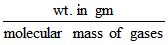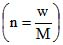Two other useful formula to calculate number of moles of gas are

(ii) number of moles =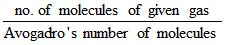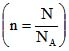(iii) no. of moles =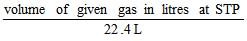When container contains more than one gas then molecular mass of mixture is termed as effective molecular mass (EMM) which is intermediate between molecular masses of all the gases present in the container.

Effective molecular mass =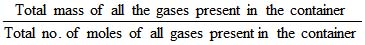2. volume

Def. Volume of gas is nothing but volume of the container in which it is present.

Relation between different units of volume

1 m3 = 103 dm3 = 103 litre =106 cm3 = 10ml = 109 mm3

3. Temperature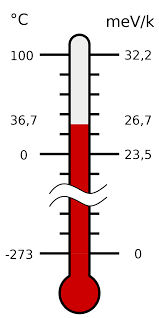Fig: Temperature calculating hotness or coldness of body

Def. Degree of  hotness or coldness of a body is measured by temperature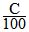=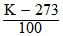=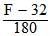C - Celsius scale, K - Kelvin scale, F - Fahrenheit scale

Note : In all the problems of gaseous state (i.e. in all gas law equations), temperature must be expressed in kelvin scale. i.e. , t °C 273 = TK

4. Pressure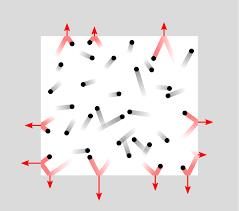Fig: Pressure is force applied to object

Def. Force acting per unit area. Pressure is the force applied perpendicular to the surface of an object per unit area over which that force is distributed.

P =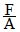units :

CGS : dyne/cm2

MKS : Newton/m2 (1N/m2 = 1Pa)

Relation : 1 N/m2 = 10 dyne/cm2

units of pressure :

1 atm = 76 cm of Hg

= 760 mm of Hg

= 760 torr

= 1.01325×105 N/m2

= 101.325 kPa

= 1.01325 bar

= 14.7 lb/In2 (Psi)

= 10.33 meters of H2O

5. Density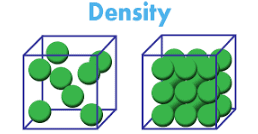Fig: Density

Def. Density is a characteristic property of a substance.

mass per unit volume

d = m/v

units :

CGS : g/cm3

mks : kg/m3

Relation : 1 kg/m3 = 10-3 g/cm3

Density of gases

Absolute density

(mass per unit volume)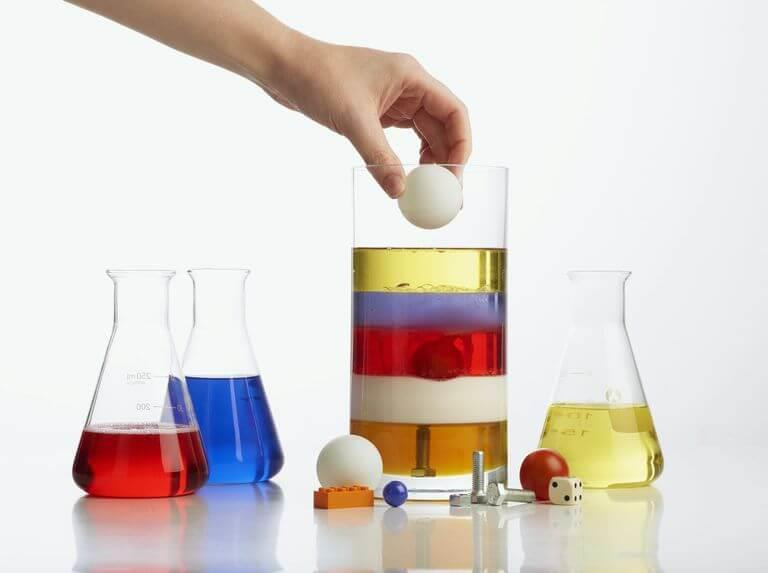Fig: Absolute density

(i) d = m/v

(ii)  unit : g/l

(iii)  function of temp., pressure, no. of moles

Relative density
(Relative to hydrogen turned as vapour density)

(i) VD =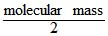(ii) No unit
(iii) independent of Pressure , Temperature

Note: mass , volume and no. of moles are extensive properties that depend on mass hence then all directly additive in nature.

Note: Density, pressure, and temperature are intensive properties they do not depend on mass hence they are non-additive in nature.

Offer running on EduRev: Apply code STAYHOME200 to get INR 200 off on our premium plan EduRev Infinity!

## Chemistry for JEE

223 videos|452 docs|334 tests

,

,

,

,

,

,

,

,

,

,

,

,

,

,

,

,

,

,

,

,

,

;